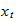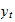StatLect

# Boosted tree

We have already learned about gradient boosting and decision trees. As a consequence, it will be easy to understand the definition of a boosted tree.

A boosted tree is an additive model obtained from a gradient boosting algorithm in which decision trees (or regression trees) are used as base learners.## Popularity

Boosted trees are deemed an essential instrument in the data scientist's toolbox.

The reason is that their predictive performance is often superior to that of other models.

In fact, boosted trees are often an important ingredient in the winning solutions of forecasting competitions such as those run on Kaggle.

## Algorithms

Two of the best algorithms used to train boosted trees are:

• LightGBM (Light Gradient Boosting Machine) by Microsoft, which is very fast and efficient (it contains some nice algorithmic innovations, based on sound math, set out in this paper).

We are going to use LightGBM in our experiments.

## Example: an artificial dataset

To show the power of boosted trees, we are going to use an artificially-generated dataset that has several challenging features:

• there are 300 correlated variables in the input vector;

• the outputis a function of only 10 of them;

• the 10 relevant inputs have:

• linear effects;

• non-linear effects (square, log, cos);

• interaction effects (products);

• threshold effects (some are relevant only if others are above threshold);

• there are 500 observations in the dataset.

Before showing the performance of boosted trees, we will show the performance of Ridge regressions and boosted linear regressions.

### Import the data and use scikit-learn to split into train-val-test (60-20-20)

We first import the data and split it into train-validation-test.

``````# Import the packages used to load and manipulate the data
import numpy as np # Numpy is a Matlab-like package for array manipulation and linear algebra
import pandas as pd # Pandas is a data-analysis and table-manipulation tool

# Import the function that performs sample splits from scikit-learn
from sklearn.model_selection import train_test_split

try:
except:
y = y.values # Transform y into a numpy array

# Print some information about the output variable
print('Class and dimension of output variable:')
print(type(y))
print(y.shape)

# Load the input variables with pandas
try:
except:
x = x.values

# Print some information about the input variables
print('Class and dimension of input variables:')
print(type(x))
print(x.shape)

# Create the training sample
x_train, x_val_test, y_train, y_val_test
= train_test_split(x, y, test_size=0.4, random_state=0)

# Split the remaining observations into validation and test
x_val, x_test, y_val, y_test
= train_test_split(x_val_test, y_val_test, test_size=0.5, random_state=0)

# Print the numerosities of the three samples
print('Numerosities of training, validation and test samples:')
print(x_train.shape, x_val.shape, x_test.shape)``````

The output is:

``````Class and dimension of output variable:
class 'numpy.ndarray'
(500, 1)
Class and dimension of input variables:
class 'numpy.ndarray'
(500, 300)
Numerosities of training, validation and test samples:
300 100 100``````

### Try with a Ridge regression

We first try to predict the new data with a Ridge regression (the regularization parameter is chosen optimally).

``````# Import functions from scikit-learn
from sklearn import linear_model # Linear regression
from sklearn.metrics import mean_squared_error, r2_score # MSE and R squared

# Create linear regression object
lr = linear_model.LinearRegression()

# Train the model using the training set
lr.fit(x_train, y_train)

# Make predictions on the training and validation sets
y_train_pred = lr.predict(x_train)
y_val_pred = lr.predict(x_val)

# Save MSE on validation set of unregularized regression
MSE = mean_squared_error(y_val, y_val_pred)

# Set up grid for regularization parameter
exponents = np.arange(1,300)
lambdas = 10 * 0.90 ** exponents

# Estimate Ridge regression for each regularization parameter in grid
# and save if performance on validation is better than that of
# previous regressions
for lambda_j in lambdas:
lr_j = linear_model.Ridge(lambda_j, normalize=True)
lr_j.fit(x_train, y_train)
y_val_pred_j = lr_j.predict(x_val)
MSE_j = mean_squared_error(y_val, y_val_pred_j)
if MSE_j < MSE:
lr = lr_j
MSE = MSE_j

# Make predictions on the train, validation and test sets
y_train_pred = lr.predict(x_train)
y_val_pred = lr.predict(x_val)
y_test_pred = lr.predict(x_test)

# Print empirical risk on all sets
print('MSE on training set:')
print(mean_squared_error(y_train, y_train_pred))
print('MSE on validation set:')
print(mean_squared_error(y_val, y_val_pred))
print('MSE on test set:')
print(mean_squared_error(y_test, y_test_pred))
print('')

# Print R squared on all sets
print('R squared on training set:')
print(r2_score(y_train, y_train_pred))
print('R squared on validation set:')
print(r2_score(y_val, y_val_pred))
print('R squared on test set:')
print(r2_score(y_test, y_test_pred))``````

The output is:

``````MSE on training set:
128.86818409871523
MSE on validation set:
253.78128412688977
MSE on test set:
216.09323621487184

R squared on training set:
0.13326769872391586
R squared on validation set:
-0.021058013938586084
R squared on test set:
-0.0289477213661109``````

Due to the highly nonlinear relationship between inputs and outputs, a linear model has no predictive ability.

### Try with a boosted linear regression

``````# Import package used to make copies of objects
from copy import deepcopy

# Our boosted linear regression (blr) class will implement 3 methods
# (constructor, fit, and predict), as previously seen in scikit-learn
class blr:
def __init__(self, learning_rate, max_iter, early_stopping):
self.lr = learning_rate
self.max_iter = max_iter
self.early = early_stopping
self.y_mean = 0
self.y_std = 1
self.x_mean = 0
self.x_std = 1
self.theta = 0
self.mses = []

def fit(self, x_train_0, y_train_0, x_val_0, y_val_0):
# Make copies of data to avoid over-writing original dataset
x_train = deepcopy(x_train_0)
y_train = deepcopy(y_train_0)
x_val = deepcopy(x_val_0)
y_val = deepcopy(y_val_0)

# De-mean the output variable
self.y_mean = np.mean(y_train)
y_train -= self.y_mean
y_val -= self.y_mean

# Standardize the output variable
self.y_std = np.std(y_train)
y_train /= self.y_std
y_val /= self.y_std

# De-mean the input variables
self.x_mean = np.mean(x_train, axis=0, keepdims=True)
x_train -= self.x_mean
x_val -= self.x_mean

# Standardize the input variables
self.x_std = np.std(x_train, axis=0, keepdims=True)
x_train /= self.x_std
x_val /= self.x_std

# Initialize counters (total boosting iterations and unproductive iterations)
current_iter = 0
no_improvement = 0

# The starting model has all coefficients equal to zero and predicts a constant zero output
self.theta = np.zeros((x_train.shape, 1))
y_train_pred = 0 * y_train
y_val_pred = 0 * y_val
eta = y_train - y_train_pred
mses = [np.var(y_val - y_val_pred)]

# Boosting iterations
while no_improvement < self.early and current_iter < self.max_iter:
current_iter += 1
corr_coeffs = np.mean(x_train * eta, axis=0) # Correlations (equal to betas) beteen residual and inputs
index_best = np.argmax(np.abs(corr_coeffs)) # Choose variable that has maximum correlation with residual
self.theta[index_best] += self.lr * corr_coeffs[index_best] # Parameter update
y_train_pred += self.lr * corr_coeffs[index_best] * x_train[:, [index_best]] # Prediction update
eta = y_train - y_train_pred # Residuals update
y_val_pred += self.lr * corr_coeffs[index_best] * x_val[:, [index_best]] # Validation prediction update
mses.append(np.var(y_val - y_val_pred)) # New validation MSE
if mses[-1] > np.min(mses[0:-1]): # Stopping criterion to avoid over-fitting
no_improvement += 1
else:
no_improvement = 0

# Final output message
print('Boosting stopped after ' + str(current_iter) + ' iterations')

def predict(self, x_test_0):
# Make copies of the data to avoid over-writing original dataset
x_test = deepcopy(x_test_0)

# De-mean input variables using means on training sample
x_test = x_test - self.x_mean

# Standardize output variables using standard deviations on training sample
x_test = x_test / self.x_std

# Return prediction
return self.y_mean + self.y_std * np.dot(x_test,self.theta)

# Create a boosted linear regression object
lr = blr(0.1, 10000, 20)

# Train the model
lr.fit(x_train, y_train, x_val, y_val)

# Make predictions on the train, validation and test sets
y_train_pred = lr.predict(x_train)
y_val_pred = lr.predict(x_val)
y_test_pred = lr.predict(x_test)

# Print empirical risk on all sets
print('MSE on training set:')
print(mean_squared_error(y_train, y_train_pred))
print('MSE on validation set:')
print(mean_squared_error(y_val, y_val_pred))
print('MSE on test set:')
print(mean_squared_error(y_test, y_test_pred))
print('')

# Print R squared on all sets
print('R squared on training set:')
print(r2_score(y_train, y_train_pred))
print('R squared on validation set:')
print(r2_score(y_val, y_val_pred))
print('R squared on test set:')
print(r2_score(y_test, y_test_pred))``````

The output is:

``````Boosting stopped after 20 iterations
MSE on training set:
139.97249675130936
MSE on validation set:
255.1793571416532
MSE on test set:
209.11804614678485

R squared on training set:
0.05858311674750227
R squared on validation set:
-0.026682990030525655
R squared on test set:
0.004265284521389967``````

Due to the highly nonlinear relationship between inputs and outputs, also the boosted linear regression has no predictive ability.

### Finally use LightGBM

We now use a boosted tree, trained with the LightGBM algorithm.

``````#Import the lightGBM package
import lightgbm as lgb

# Prepare dataset in LightGMB format
y_train = np.squeeze(y_train)
y_val = np.squeeze(y_val)
y_test = np.squeeze(y_test)
train_set = lgb.Dataset(x_train, y_train, silent=True)
valid_set = lgb.Dataset(x_val, y_val, silent=True)

# Set some algorithm parameters
params = {
'objective': 'regression',
'learning_rate': 0.1,
'metric': 'mse',
'min_data_in_leaf': 10,
'max_depth': 2,
}

# Train the model
boosted_tree = lgb.train(
params = params,
train_set = train_set,
valid_sets = valid_set,
num_boost_round = 10000,
early_stopping_rounds = 20,
verbose_eval = -1,
)

# Make predictions on the train, validation and test sets
y_train_pred = boosted_tree.predict(x_train)
y_val_pred = boosted_tree.predict(x_val)
y_test_pred = boosted_tree.predict(x_test)

# Print empirical risk on all sets
print('')
print('MSE on training set:')
print(mean_squared_error(y_train, y_train_pred))
print('MSE on validation set:')
print(mean_squared_error(y_val, y_val_pred))
print('MSE on test set:')
print(mean_squared_error(y_test, y_test_pred))
print('')

# Print R squared on all sets
print('R squared on training set:')
print(r2_score(y_train, y_train_pred))
print('R squared on validation set:')
print(r2_score(y_val, y_val_pred))
print('R squared on test set:')
print(r2_score(y_test, y_test_pred))``````

The output is:

``````Training until validation scores don't improve for 20 rounds
Early stopping, best iteration is:
	valid_0's l2: 59.4414

MSE on training set:
4.51945627370331
MSE on validation set:
59.44138540489672
MSE on test set:
55.648549144794714

R squared on training set:
0.9696033682477989
R squared on validation set:
0.760844842691648
R squared on test set:
0.7350243402207612``````

This is quite an impressive result! The predictive model generated by LightGBM produces fairly accurate forecasts. It is able to:

• correctly capture the nonlinearity in the data;

• deal with the large number of irrelevant input variables.

### Now add lots of missing values and see what happens

We now add some missing values and see how the algorithm performs.

``````# Set seed for random numbel generation
np.random.seed(0)

# Create function that randomly replaces a given proportion of values with nans
def drop_values(np_array, proportion_of_missing):
n_values = np_array.size
n_to_replace = int(np.floor(np_array.size * proportion_of_missing))
indices = np.random.choice(n_values, n_to_replace, replace=False)
np_array.ravel()[indices] = np.nan

# Replace 20 per cent of the entries of the input matrices with missing values
drop_values(x_train, 0.1)
drop_values(x_val, 0.1)
drop_values(x_test, 0.1)

# Prepare dataset in LightGMB format
train_set = lgb.Dataset(x_train, y_train, silent=True)
valid_set = lgb.Dataset(x_val, y_val, silent=True)

# Set some algorithm parameters
params = {
'objective': 'regression',
'learning_rate': 0.1,
'metric': 'mse',
'min_data_in_leaf': 10,
'max_depth': 2,
}

# Train the model
boosted_tree = lgb.train(
params = params,
train_set = train_set,
valid_sets = valid_set,
num_boost_round = 10000,
early_stopping_rounds = 20,
verbose_eval = -1,
)

# Make predictions on the train, validation and test sets
y_train_pred = boosted_tree.predict(x_train)
y_val_pred = boosted_tree.predict(x_val)
y_test_pred = boosted_tree.predict(x_test)

# Print empirical risk on all sets
print('')
print('MSE on training set:')
print(mean_squared_error(y_train, y_train_pred))
print('MSE on validation set:')
print(mean_squared_error(y_val, y_val_pred))
print('MSE on test set:')
print(mean_squared_error(y_test, y_test_pred))
print('')

# Print R squared on all sets
print('R squared on training set:')
print(r2_score(y_train, y_train_pred))
print('R squared on validation set:')
print(r2_score(y_val, y_val_pred))
print('R squared on test set:')
print(r2_score(y_test, y_test_pred))``````

The output is:

``````Training until validation scores don't improve for 20 rounds
Early stopping, best iteration is:
	valid_0's l2: 78.2192

MSE on training set:
1.9500449940783635
MSE on validation set:
78.21919805435142
MSE on test set:
67.23087205915962

R squared on training set:
0.986884528581432
R squared on validation set:
0.6852946059753087
R squared on test set:
0.6798740496350253``````

Wow! The predictive performance of the model was affected only marginally by the large number of missing values.